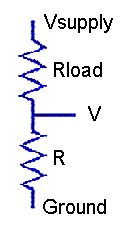DAPL Operating System | Processing Command

Module SENSORM :: DIVIDER

Convert measurements from a voltage divider network to determine resistance.

Syntax

DIVIDER( VIN, VS, RLOAD, [RCOEFF, LTMP,] [GAIN,] ROUT )

Parameters

VIN
Input measurements of divider voltage
FLOAT PIPE
VS
Nominal or measured excitation voltage driving divider network
FLOAT CONSTANT | FLOAT PIPE
Nominal or calibrated load resistance, ohms at 0 degrees C
FLOAT CONSTANT
RCOEFF
Temperature coefficient of resistance for load resistor
FLOAT CONSTANT
LTMP
Input pipe with load temperature measurements, degrees C
FLOAT PIPE
GAIN
Gain used to measure input signal `VIN`
FLOAT CONSTANT
ROUT
Output resistance measurements, in ohms
FLOAT PIPE

Description

The ` DIVIDER ` command converts voltage readings to measure an unknown resistance. The unknown device to be measured is placed in a voltage divider network with an accurately-known loading resistor. The divider network is driven by an accurately-regulated voltage source, and the voltages ` VIN ` are observed at the junction between the unknown element and load resistor. The results, in ohms, are returned through the ` ROUT ` pipe, one result per divider measurement input value.The input divider voltage measurements are received through the ` VIN ` pipe. These measurements have units of volts. By default, this command assumes that the voltage measurement are obtained using an amplifier gain of 1.0, but if you use a higher gain, specify it as the ` GAIN ` parameter.

If the supply voltage is very well regulated, you can measure it once and specify the value as a constant ``` VS ``` parameter. If you use the regulated voltage from the data acquisition processor for this excitation, you could specify 5.0 volts and omit the calibration measurement. If the excitation is subject to small but potentially relevant variations, make simultaneous voltage measurements of the supply voltage, in units of volts, and provide this second input stream to the ` DIVIDER ` command through the ``` VS ``` pipe, one supply voltage measurement per divider voltage reading.

Specify the loading resistor value ` RLOAD ` parameter in units of ohms. Sometimes a nominal resistor value is close enough, but for better results enter an accurately- measured value.

Most of the time, variations in operating temperature are insignificant, and the load resistance can be presumed constant. For these cases, omit the ` RCOEFF ` and ``` LTMP``` parameters. However, if significant temperature variations are anticipated, the ` DIVIDER ` command can adjust the effective load resistance to compensate for temperature-dependent changes. Set the ` RCOEFF ` parameter to the temperature coefficient of load resistance in ohms per degree Centigrade. Adjust the ` RLOAD ` parameter if necessary so that it reports the correct loading resistance at 0 degrees Centigrade. Measure the load resistance temperature in units of degrees Centigrade and send these measurements to the ` DIVIDER ` command through the ` LTMP ` pipe, one temperature reading per divider measurement.

For processing each input value, the difference between the known excitation voltage source and the measured divider junction appears across the known loading resistor, hence the divider current can be computed. This known divider current passes through the unknown element to produce the measured voltage, so its resistance can be computed, producing the results placed into the ` ROUT ` pipe.

Examples

```  DIVIDER(IP2, 5.000, 10000.0, ROUT)
```

Read the voltage at the junction between a load resistance of exactly 10K ohms and an unknown resistive sensor when a 5.000 volt excitation voltage is applied across the divider network. The voltages across the resistive sensor element are measured with gain 1 and received from input sample channel pipe `IPipe 2. ` The computed resistance values are reported in pipe ` ROUT. `

```  DIVIDER(IP2, 4.968, 10008.0, 0.42, PAmb, 4.0, ROUT)
```

This example is the same as the previous configuration, except calibrated for maximum measurement accuracy. The pre-measured supply voltage level is 4.958 ohms is specified as a constant. The measured load resistor value is 10008 ohms. The nominal load resistance is observed to increase by 42 ohms over a 100 degree temperature swing, so a temperature coefficient 0.42 ohms per degrees C is specified. The measurements of divider voltage are captured using an amplifier gain of 4.0. The operating temperature is separately measured and provided in the `PAmb` pipe. The computations use a temperature-adjusted value of load resistance for each conversion.

` BRIDGE `